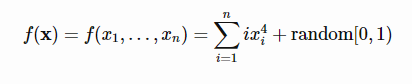top of page
Search

# QUARTIC FUNCTION

Updated: Jul 18, 2021Mathematical DefinitionInput Domain

The function can be defined on any input domain but it is usually evaluated on xi ∈[−1.28,1.28] for i=1,…,n. Here, n = 2.

Global Minima

The function has one global minimum f(x*)=0+random noise at x*=(0,…,0).

Description and Features

The function is continuous, not convex, defined on n-dimensional space, multimodal, differentiable, separable.

Python Implementation

```% Please forward any comments or bug reports in chat
Copyright 2021. INDUSMIC PRIVATE LIMITED.THERE IS NO WARRANTY, EXPRESS OR IMPLIED. WE DO NOT ASSUME ANY LIABILITY FOR THE USE OF THIS PROGRAM. If software is modified to produce derivative works, such modified software should be clearly marked. Additionally, user can redistribute it and/or modify it under the terms of the GNU General Public License. This program is distributed in the hope that it will be useful, but WITHOUT ANY WARRANTY. See the GNU General Public License for more details.
% for any support connect with us on help.indusmic@gmail.com

import numpy as np
import matplotlib.pyplot as plt
from numpy import random
fig = plt.figure()
ax = fig.gca(projection='3d')
x = np.arange(-1.28, 1.28,0.1)
y = np.arange(-1.28, 1.28, 0.1)
x, y = np.meshgrid(x, y)
z = random.randint(0,1)
sum =0
for i in range(3):
sum = sum + (i * (x**4 + y **4))
result = sum + z
surface = ax.plot_surface(x, y, result, cmap='jet')
plt.show()
plt.contour(x,y,result)
plt.show()
plt.scatter(x, y, result)
plt.show()```

References:

 Jamil, Momin, and Xin-She Yang. "A literature survey of benchmark functions for global optimization problems." International Journal of Mathematical Modelling and Numerical Optimization 4.2 (2013): 150-194.